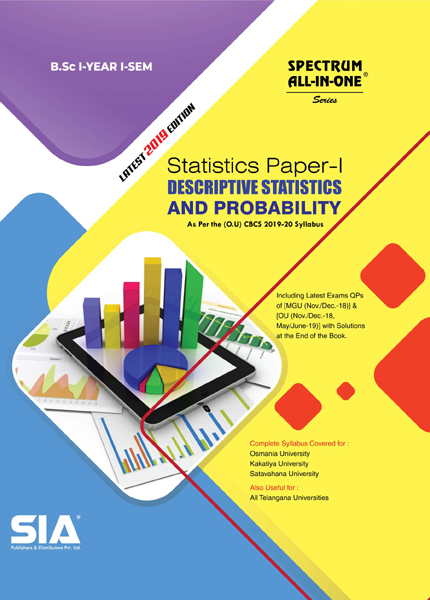•My WalletMy Order
•My Profile
•My Connections
•My Books
•My Videos
•My Tests
•My Calender
•My Messages
•My Shopping Cart
•My Orders
•Account Settings
•Help

# Book Details# Descriptive Statistics Probability (Statistics Paper-I)

 Course Code : B.Sc I-I (O.U) Author : SIA PUBLISHERS University : Osmania University Regulation : 2019 Categories : Arts and Science Format :PDF (DRM Protected) Type : eBook

Rs.220 Rs.69 Rs.69% off

Description :

UNIT-I

Descriptive Statistics: Concept of Primary and Secondary Data. Methods of Collection and Editing of Primary Data. Designing a Questionnaire and a Schedule. Sources and Editing of Secondary Data. Classification and Tabulation of Data. Measures of Central Tendency (Arithmetic Mean, Median, Mode, Geometric Mean and Harmonic Mean) with Simple Applications. Absolute and Relative Measures of Dispersion (Range, Quartile Deviation, Mean Deviation, Standard Deviation) with Simple Applications, Importance of Moments, Central and Non-central Moments, their Interrelationships, Sheppard’s Corrections for Moments for Grouped Data. Measures of Skewness Based on Quartiles and Moments, Kurtosis Based on Moments with Real Life Examples.

UNIT-II

Probability: Basic Concepts in Probability, Deterministic and Random Experiments, Trial, Outcome, Sample Space, Event and Operations of Events, Mutually Exclusive and Exhaustive

Events, Equally Likely and Favourable Outcomes with Examples. Mathematical, Statistical and Axiomatic Definitions of Probability with Merits and Demerits. Properties of Probability Based on Axiomatic Definition. Conditional Probability and Independence of Events, Addition and Multiplication Theorems for n Events. Boole’s Inequality and Bayes’ Theorem. Problems on Probability using Counting Methods and Theorems.

UNIT-III

Random Variables: Definition of Random Variable, Discrete and Continuous Random Variables, Functions of Random Variables, Probability Mass Function and Probability Density

Function with Illustrations. Distribution Function and its Properties. Transformation of Onedimensional Random Variable (Simple 1-1 Functions Only). Notion of Bivariate Random Variable, Bivariate Distribution, Statements of its Properties. Joint, Marginal and Conditional Distributions, Independence of Random Variables.

UNIT-IV

Mathematical Expectation: Mathematical Expectation of a Function of a Random Variable, Raw and Central Moments, Covariance Using Mathematical Expectation with Examples,

Addition and Multiplication Theorems of Expectation. Definitions of Moment Generating Function (m.g.f), Characteristic Function (c.f), Cumulant Generating Function (c.g.f), Probability Generating Function (p.g.f) and Statements of their Properties with Applications. Chebyshev’s and Cauchy-Schwartz’s Inequalities and their Applications.

Note : Descriptive Statistics Probability (Statistics Paper-I), B.Sc I-I (O.U)

##### Publisher Detail:

Publisher Name: SIA Publishers and Distributors (P) Ltd.

Contact Email Id: msultan@siaedugroup.com